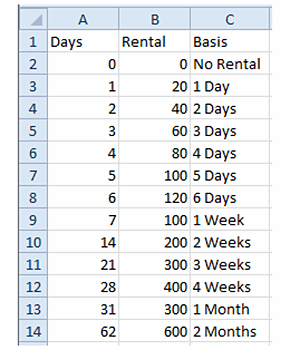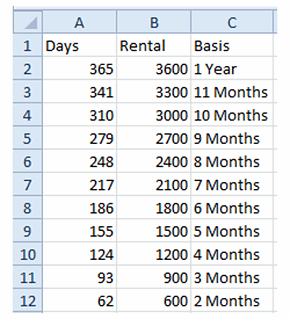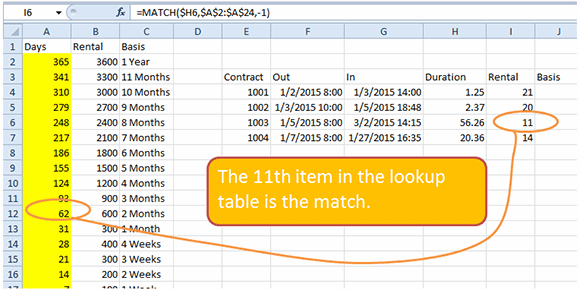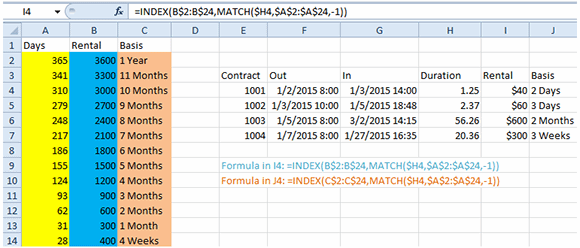This content is part of the Essential Guide: A guide to using Excel as financial accounting software

### Essential Guide

Browse Sections
• Managing financial data in Excel -- and beyond
• Excel's changing role in the enterprise
• Must-know terms for accounting with Excel

# Finding the next largest match with the Excel MATCH function

## Microsoft Excel consultant Bill Jelen, aka MrExcel, walks readers through an exercise on using the MATCH function.

The VLOOKUP function in Microsoft Excel is great at finding exact matches or the value just less than the lookup...

value. However, in certain situations, you might need to find the next largest record. VLOOKUP cannot do this, but the MATCH function can.

Say that you work for a rental company. Rental periods for 0 through 6 days are rounded up to the next highest day. Rental periods for days 7 through 28 are rounded up to the next highest week. Rental periods for 29 through 365 days are rounded to the next highest month.

You might initially set up a range lookup table such as this one:Figure 1

However, for this trick to work, the table needs to be sorted in descending order, as shown here:Figure 2

Instead of VLOOKUP, you will use the MATCH function. While most people have not used MATCH, it is very similar to VLOOKUP. The lookup value is the same as VLOOKUP. The lookup table has to be only a single row or column instead of a rectangular table. You don't have to specify the column number. The last argument can be 0 (equivalent to FALSE in VLOOKUP), 1 (equivalent to TRUE in VLOOKUP) or -1 (no equivalent in VLOOKUP -- returns a value just larger).

Here is the oddity: MATCH returns the relative position of the matching row. In Figure 3, the formula =MATCH(H6,A2:A24,-1) in cell I6 returns an 11 because the matching row is in A12, which is the 11th item in the lookup range.Figure 3

You might wonder, what good is knowing that the match is in the 11th cell in the range? You need to use the MATCH as the second argument in the INDEX function.

The INDEX function takes a range of possible answers and an index number. =INDEX(B2:B24,11) would return the value from B12. =INDEX(B2:B24,MATCH(H6,A2:A24,-1) returns the Rental value. In order to make the formula able to be copied, use = INDEX(B\$2:B\$24,MATCH(H6,\$A\$2:\$A\$24,-1) in I4 and copy to I4:J6.Figure 4